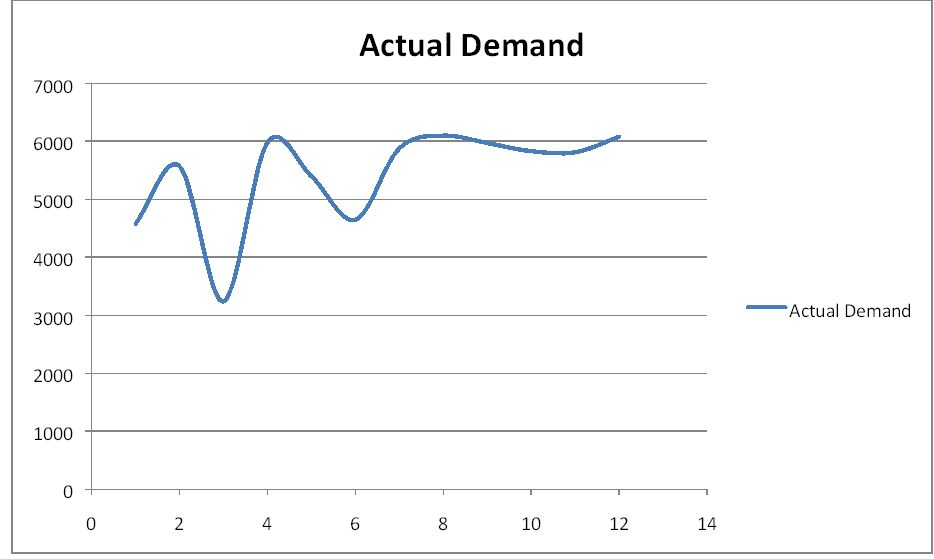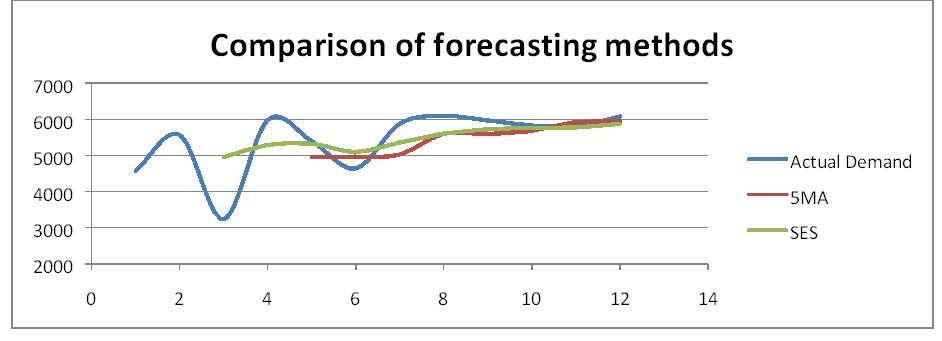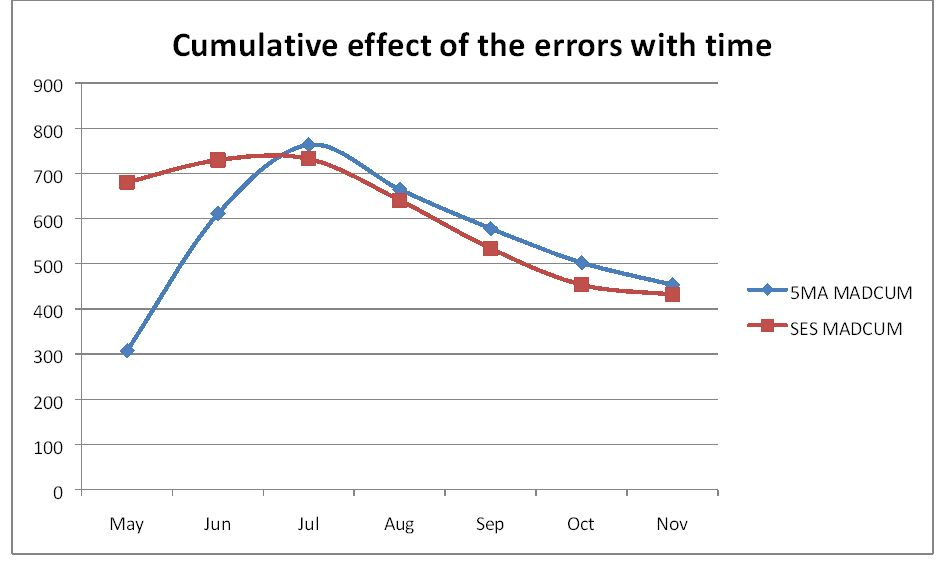# Choice of moving average or exponential smoothing for a particular product profile

article
product
excel
forecasting
Published

November 23, 2015

Modified

November 23, 2015

## Introduction

The demand data for a product has been shown in the table below. Compare the forecasts using a Moving Average with a period of 5 months, MA(5), and an Exponential smoothing Method with an α of 0.33. For Exponential Smoothing use the midpoint of first 5 month range of the average as the initial Forecast. (Hint: the Exponential Smoothing Forecast will be initialized with a forecast of 4951 for April made in March.)

## Visualization of raw data### Forecasting with 5-point moving average & simple exponential smoothing

Here we use α=1/3 for our exponential smoothing model### Accuracy of the models

To estimate the accuracy of the models, we first compare the Mean Absolute Deviation (MAD) for each of the models.

5MA SES